Next: 6.2 Simulation Procedure Up: 6. Simulation of Thermal Previous: 6. Simulation of Thermal

Subsections

# 6.1 Architecture of FEDOS

The core functions of FEDOS are the management of the simulation procedure and data flow, the model execution, and the finite element assembling. Some functions like data- and Inputdeck-file operations or the solving of the equation system are provided by libraries, but these function calls are incumbent on FEDOS. Furthermore, FEDOS offers a number of operations for mesh manipulation, especially for (dynamic) mesh refinement and coarsement.

## 6.1.1 Inputdeck

FEDOS always asks for a so-called Inputdeck-file (ipd-file) which includes all necessary information for a simulation run. In principle the ipd-file contains all changeable process information. The Inputdeck-file can be read with the Inputdeck-Reader which is a library linked into FEDOS. The Inputdeck concept was also developed at the Institute for Microelectronics (see Chapter 3 in ) and is also used for other simulators. The ipd-file itself is an Ascii-file which can be generated with a normal text editor in an evident syntax.

The ipd-file includes the names of the input and the output file. Alternatively sometimes it is desired to set an attribute to a constant initial value on the whole segment. In the case of oxidation simulation the normalized silicon concentrationmust be set to the initial value 1 in the silicon segment (see Section 3.1). The next important task is to control the simulation procedure which involves amongst others the

• Maximal time of the simulated process
• Duration of one time step
• Number of time steps
• If the duration of the time steps is constant or increased recursively
In the ipd-file also the accuracy for the Newton solver is determined (see Section 5.3.5) which is related with the controlling part. For a desired higher accuracy of the results more Newton loops and so more simulation time is needed for solving a non-linear equation system.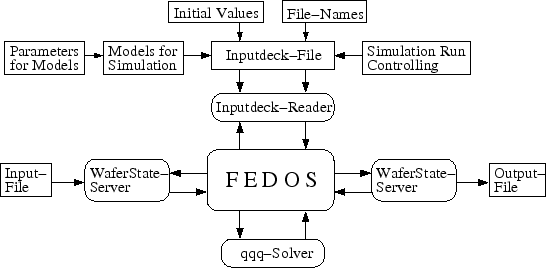Since FEDOS contains a number of different models, another necessary part in the ipd-file is to declare which model is applied on the respective segment by its name. The models can be divided into the three categories:

• Volume models: describe the physical behavior within a segment. Examples are the models for oxidation, diffusion, or mechanics. Furthermore, it is obligatory to assign a volume model to each segment. This means that also on not relevant segments of a more complex simulation setup a dummy model must be applied.
• Surface models: can be applied on segment surfaces with boundary conditions. The surface models always contain a Dirichlet or Neumann boundary condition. Boundary conditions for the mechanics (Dirichlet) or the species flow for diffusion (Neumann) can be listed. On surfaces without explicit models FEM assumes implicitly a Neumann boundary condition which means that there exists no flow of particles through the surface. In contrast to the volume models the mathematical formulation in the surface model has to be performed for triangles, because a surface only has two dimensions.
• Interface models: describe the physical behavior on the interface between two adjacent segments. Like the surface also an interface only has two dimensions. As depicted in Section 5.3.3 an interface model is essential for mechanical problems. Another example is the segregation of species at an interface.
Simulation only makes sense, when the process parameters are changeable and so at least all volume models have its own parameters. For the oxidation model such parameters are the low stress diffusion coefficient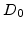(see (3.2)) or the maximal strength of the spatial sink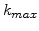(see (3.4)). In the mechanical models the Young modulus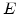and the Poisson ratio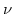are modifiable.

## 6.1.2 Wafer-State-Server

For the data management FEDOS uses the WAFER-STATE-SERVER , a program package developed at the Institute for Microelectronics. All data are saved in the so-called WAFER-STATE-SERVER-file (WSS-file) in an Ascii-format. The WSS-format enables straightforward communication of FEDOS with other in-house tools as for meshing of the structure or visualization of the simulation results.

In the WSS-file are one or more segments where each segment holds a (tetrahedral) grid. On the segment grid a unlimited number of constant or distributed attributes can be located. The WSS-file concept has the benefit regarding the file size that the coordinates (x-, y- and z-value) of each grid point are only saved once although, a grid point is shared by a number of tetrahedrons. Therefore, the nodes of the tetrahedrons in the segment grid are only references to a point list. Another memory saving effect is that the distributed attribute values are also saved only once on the grid points in the respective segment and not on each tetrahedron node.

The WAFER-STATE-SERVER is not merely a file reading and writing tool, it is in principle a data management tool. In the beginning all grid and attribute information from the input file are read and then held in the WAFER-STATE-SERVER during the simulation. It achieves an abstraction of the physical stored data in the file to logical dats in the program. For FEDOS the WAFER-STATE-SERVER supplies a lot of useful grid operations like surface and interface extraction, point and element location, or attribute updates during the simulation. For simulation with FEDOS the WSS input file must at least contain the grid information of the discretized structure. The simulation results are written to the output file in form of distributed attributes. For the oxidation simulation the results are the distribution of the oxidant concentration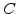and the normalized silicon concentration. For the mechanical problem with its displacements also the point coordinates are modified in the output file.

## 6.1.3 QQQ-solver

The solving of the (linear) global equation system is performed with the QQQ-solver , also developed at the Institute for Microelectronics. The QQQ-solver is based on the Gaussian method , which uses a factorization of the matrix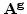in a lower and upper triangular matrix (), so that the equation system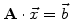can be written as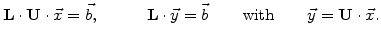(6.1)

Therefore, the Gaussian algorithm is specified by the following three steps:

1.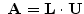: Gaussian elimination by factorization (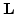and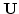is computed )

2.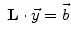: forward-substitution (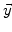is computed )

3.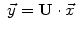: backward-substitution (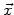is computed )

The QQQ-solver also supplies a transformation matrix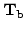which allows to transform the equation systemto(6.2)

As depicted in Section 5.3.2 and 5.3.3 the matrixcan be used for the elimination of equations not needed because of Dirichlet boundary conditions or for correcting the equation system in case of mechanical interfaces.

The assembling of the equation system is performed by FEDOS by generating the matrices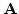,and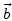for the QQQ-module. After solving the QQQ-module returns the results to FEDOS. The complete equation system for the oxidation problem (see Section 5.3.4) consists of the non-linear (diffusion-reaction) part and the linear (mechanical) part.

The non-linear sub-system requires some Newton iterations, until it fulfills the termination conditions. It should be mentioned that the QQQ-module is not a non-linear solver, it can only can handle linear systems. As described in Section 5.3.5 FEDOS assembles the non-linear sub-system in such a kind that it becomes a linear system for increments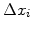, which can be solved by the QQQ-module. These increments are computed in a way that FEDOS can build a solution. This procedure is repeated until the approximation fulfills the desired terminating conditions.Next: 6.2 Simulation Procedure Up: 6. Simulation of Thermal Previous: 6. Simulation of Thermal

Ch. Hollauer: Modeling of Thermal Oxidation and Stress Effects